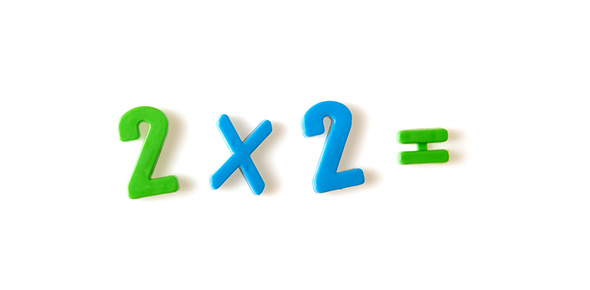# How Much Do You Know About Pi Quiz ?

10 Questions | Attempts: 961
ShareSettings'Pi' (π) is a mathematical constant whose approximate value is 3. 14159 which has been known for 4,000 years and discovered by ancient Babylonians. The Egyptians calculated the area of a circle by a formula giving 'Pi' an approximate How well do you about Pi? value. This quiz has been developed to test your knowledge about Pi and its digit functions. Play this pi symbol quiz with your friends and family members for more learning! So, let's try out the quiz. All the best!

• 1.
What are the first four digits of Pi after the decimal point?
• A.

1415

• B.

3256

• C.

2917

• D.

5971

• E.

6355

• 2.
Which four digits come after 3.1415 in Pi?
• A.

5692

• B.

1234

• C.

8264

• D.

9265

• E.

7539

• 3.
What is the oldest document known relating to the existence of Pi?
• A.

The Suse tablette from the Babylonians

• B.

The Rhind papyrus from the Egyptians

• C.

Paper on the method of Archimedes

• D.

A carved stone

• 4.
Simon Plouffe, the famous Canadian mathematician who discovered the BBP formula allowing to calculate the n-th digit of Pi in base 2, was already famous in 1975 in the Guinness record book. What for?
• A.

He calculated 2 million decimals on a Cray

• B.

He memorised 4096 decimals of π

• C.

He cooked the biggest Apple Pie (3m in circumference)

• D.

By traveling 314 km on his hands

• 5.
What is the actual record of a number of decimals calculated? (ouch, this question will be quickly obsolete, so let us indicate the date as 20th September 1999)
• A.

206,158,430,000

• B.

206,000,000,000

• C.

68,719,470,000

• D.

6,442,450,938

• 6.
In 1997 Fabrice Bellard found a formula that accelerated the calculation of the n-th digit of Pi compared to the BBP formula. By how much?
• A.

16%

• B.

27%

• C.

43%

• D.

52%

• 7.
How are the first few digits of Pi written in binary?
• A.

11.1110111101111100

• B.

1.10111011010010011

• C.

101.110101000111100

• D.

11.0010010000111111

• 8.
The definitions of Pi through geometry because of the Euclidian geometry and integral calculation from below. Mathematicians now go for analytical definitions a lot more abstract. Notably, the famous paper of Bourbaki Pi is defined as:
• A.

The constant such that exp(i*π)=-1

• B.

The double of the unique root to the equation cos(x)=0 between 0 and 2

• C.

The real number that appears in the differention 2*π*e(x) of the function e(x), unique continu homorphism of the additive group R on the multiplicative group U of the complex number of absolute number 1

• D.

The perimeter of an apple π divided by it's diameter

• 9.
Another important concept, the universal number. We don't know if Pi is one. What practical consequence could this have if the hypothesis were checked?
• A.

We could find the Bible and our own biography at a precise place in the decimals of π translate in letters

• B.

We could calculate the size of the universe

• C.

We could finally have a discussion with π

• D.

We won't need to calculate it's decimal anymore because we could guess them using the previous one

• 10.
A contemporary mathematician and who participated in the research of Pi owned a number plate whose number was P 314159. Nowadays it's in hexadecimal, who was it?
• A.

Simon Plouffe

• B.

David Bailey

• C.

Peter Borwein

• D.

David Chudnovsky

## Related TopicsBack to top
×

Wait!
Here's an interesting quiz for you.